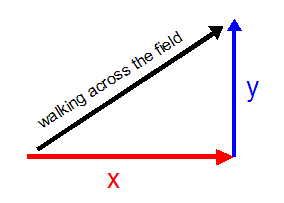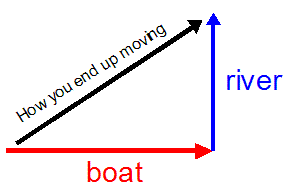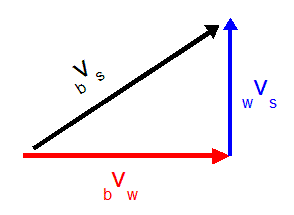Lesson 23: Components of Vectors

The idea of components of vectors lets you do all sorts of questions that would be impossible otherwise.

• Component just means "parts".
• You might have a component stereo system, which just means it is made up of parts (amplifier, tuner, equalizer, cd player, etc.) that all work together as one whole thing (a stereo).

Components of vectors are just two perpendicular vectors that added together would give you the original vector.

• As a general rule the components should always be perpendicular (which is why they are sometimes called orthogonal components).
• Usually they are called either x and y or horizontal and vertical.
• The components are mutually independent, which is just a fancy way of saying that if one of them changes it will not affect the other. For example, if x got bigger, y would still be the same. Only the resultant would change.

The following image shows how walking across a field diagonally could be broken into x and y components.Figure 1
• You can see here how the x and y vectors added together give us the resultant of actually walking across the field diagonally.
• If the person walked along x and then y it would end up the same way.

## Using Components to Solve Problems

If you've ever done any boating on a fast moving river, you know how difficult it can be to get where you want to go.Figure 2
• Let's say you point your boat directly East across a river that is flowing North.
• As your boat moves itself through the water towards the East, the river is constantly pushing it to the North.
• In the end someone watching from the shore would say that you were moving in a direction roughly North-East.
• Components will either let you figure out how the boat ends up moving, or take how the boat is moving apart into components.

Note: Calling the vectors above "boat" , "river", and "how you end up moving" is not really accurate enough for the kinds of questions we will be looking at in detail. The following examples will show you one way to label the vectors, but you may also use any (reasonable) system of your own that you feel comfortable with.

Example 1: You are in a boat that can move in still water at 7.0 m/s. You point your boat directly East across a river to get to the other side that is 200 m away. The river is flowing at 4.0 m/s [N].

a) Determine your velocity measured by someone on the shore.To keep track of the velocities we will be dealing with we will use some subscripts:

bvw = velocity of the boat in still water

wvs = velocity of the water with respect to the shore

bvs = velocity of the boat with respect to the shore

The question is asking for the black vector called bvs, which is the resultant of two components.

c2 = a2 + b2 =
= 7.0 2 + 4.0 2
c = 8.1 m/s = bvs

tanΘ = opp/adj = wvs / bvw = 4.0 / 7.0
Θ = 30°

The boat is moving at 8.1 m/s [E30°N].

b) Determine how much time it takes for the boat to cross the river.

You must choose vectors that point in exactly the same direction to solve questions like this!

Do not be tempted to use your answer from part (a) unless you have good reason to! If you think about it, this boat is going across a river that is 200 m wide measured straight across.

Which vector is pointing straight across the river?

The red one showing the velocity of the boat as though it was moving in still water. Even though it gets pushed off course by the water, the components are mutually independent! The boat is still moving East across that river at 7.0 m/s. The displacement the boat has to travel (200 m [E]) and the velocity bvw (7.0 m/s [E] ) are the only ones that point in exactly the same direction.

v = d / t
t = d / bvw
= 200 / 7.0
t = 29 s

The boat takes 29 s to cross the river.

c) Determine how far downstream from directly across the river the boat will hit shore.

Which velocity is pushing the boat downstream? That has to be wvs. And from the last question we know how long the boat will be on the water to be able to be pushed downstream.

v = d / t
d = wvs t
= 4.0 (29)
d = 1.1e2 m

The boat will hit the shore 1.1e2 m [N] of where it was originally pointed at.

If you look back at the answer for (a) in the previous example, you should probably be able to see how giving you the velocity of 8.1 m/s [E30°N] measured by someone on the shore could be broken into components.

• This way you can figure out the velocity of the water(wvs) and the velocity of the boat in still water (bvw).
• You can either put components together to get a resultant, or break a resultant apart to get components depending on your needs.

The other popular type of question to do with components is a plane flying with a wind blowing it off course.

• You will find examples of these types of questions in the worksheet that follows this lesson.

Watch a video of me showing an example of solving components questions by clicking here. Requires Windows Media Player 9 or later and a broadband connection (dial-up connection not recommended).